# How to Calculate and Solve for Viscosity | Ceramics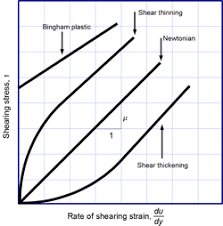The image above represents viscosity.

To compute for viscosity, three essential parameters are needed and these parameters are Force applied (F), Area (A) and Derivation Ratio of Velocity to Distance of Fluid Flow (dv/dy).

The formula for calculating viscosity:

η = F/A / dv/dy

Where:

η = Viscosity
F = Force Applied
A = Area
dv/dy = Derivation Ratio of Velocity to Distance of Fluid Flow

Let’s solve an example;
Find the viscosity when the force applied is 21, area is 14 and derivation ratio of velocity to distance of fluid flow is 19.

This implies that;

F = Force Applied = 21
A = Area = 14
dv/dy = Derivation Ratio of Velocity to Distance of Fluid Flow = 19

η = F/A / dv/dy
η = (21/14) / (19)
η = (1.5) / (19)
η = 0.0789

Therefore, the viscosity is 0.0789 Pa s.

Calculating Force Applied when the Viscosity, the Area and the Derivation ratio of velocity to distance of fluid flow is Given.

F = (η x dv/dy) A

Where;

F = Force Applied
η = Viscosity
A = Area
dv/dy = Derivation Ratio of Velocity to Distance of Fluid Flow

Let’s solve an example;
Find the force applied when the viscosity is 20, the area is 30 and the derivation is 8.

This implies that;

η = Viscosity = 20
A = Area = 30
dv/dy = Derivation Ratio of Velocity to Distance of Fluid Flow = 8

F = (η x dv/dy) A
F = (20 x 8) 30
F = (160) 30
F = 4800

Therefore, the force applied is 4800.

Calculating the Area when the Viscosity, the Force Applied and the Derivation Ratio of Velocity to Distance of Fluid Flow is Given.

A = (η x dv/dy) F

Where;

A = Area
η = Viscosity
F = Force Applied
dv/dy = Derivation Ratio of Velocity to Distance of Fluid Flow

Let’s solve an example;
Find the area when the viscosity is 12, the force applied is 9 and the derivation is 10.

This implies that;

η = Viscosity = 12
F = Force Applied = 9
dv/dy = Derivation Ratio of Velocity to Distance of Fluid Flow = 10

A = (η x dv/dy) F
A = (12 x 10) 9
A = (120) 9
A = 1080

Therefore, the area is 1080.

Calculating the Derivation Ratio of Velocity to Distance of Fluid Flow when the Viscosity, the Force Applied and the Area is Given.

dv/dy = F/A / η

Where;

dv/dy = Derivation Ratio of Velocity to Distance of Fluid Flow
η = Viscosity
F = Force Applied
A = Area

Let’s solve an example;
Find the derivation when the viscosity is 40, the force applied is 10 and the area is 2.

This implies that;

η = Viscosity = 40
F = Force Applied = 10
A = Area = 2

dv/dy = F/A / η
dv/dy = 10/2 / 40
dv/dy = 5 / 40
dv/dy = 0.125

Therefore, the derivation is 0.125.

Nickzom Calculator – The Calculator Encyclopedia is capable of calculating the viscosity.

To get the answer and workings of the viscosity using the Nickzom Calculator – The Calculator Encyclopedia. First, you need to obtain the app.

You can get this app via any of these means:

To get access to the professional version via web, you need to register and subscribe for NGN 1,500 per annum to have utter access to all functionalities.
You can also try the demo version via https://www.nickzom.org/calculator

Apple (Paid) – https://itunes.apple.com/us/app/nickzom-calculator/id1331162702?mt=8
Once, you have obtained the calculator encyclopedia app, proceed to the Calculator Map, then click on Materials & Metallurgical under Engineering.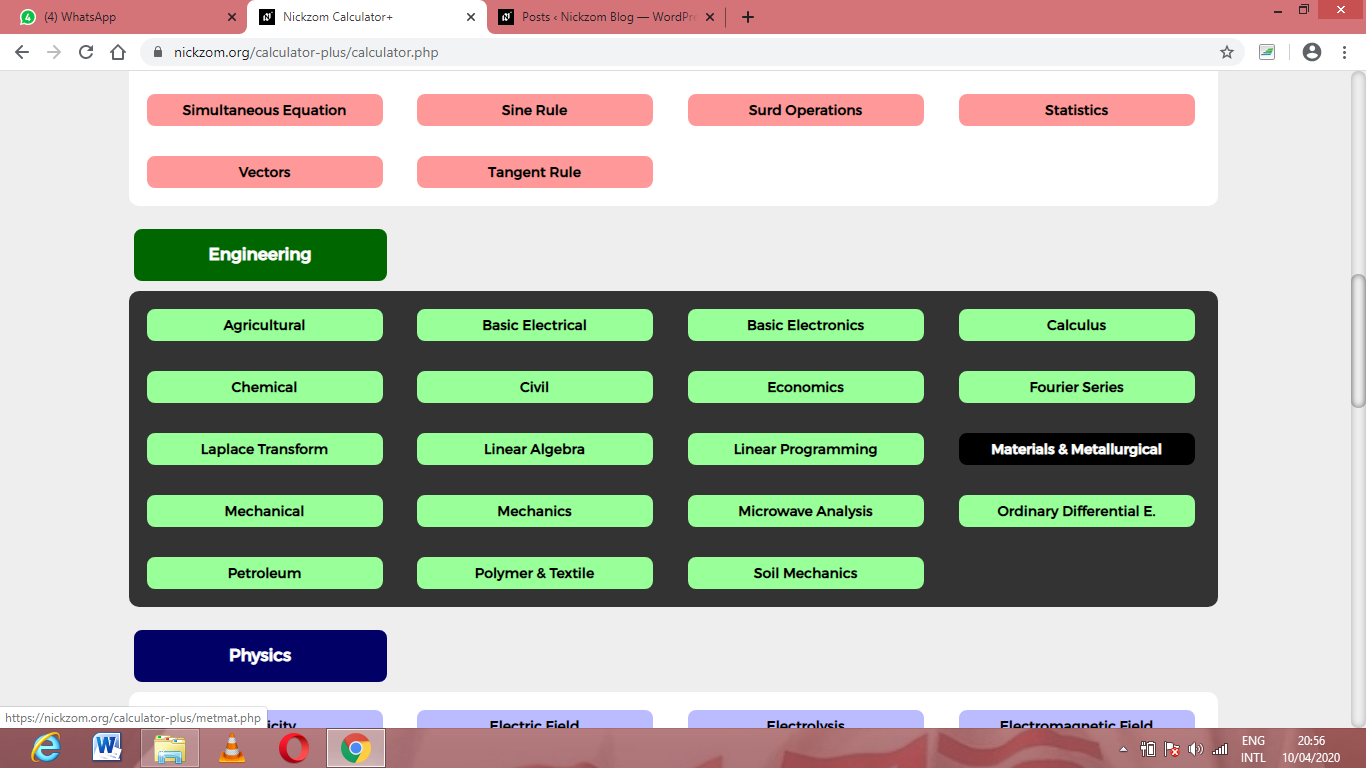Now, Click on Ceramics under Material & MetallurgicalNow, Click on Viscosity under CeramicsThe screenshot below displays the page or activity to enter your values, to get the answer for the viscosity according to the respective parameters which are the Force applied (F), Area (A) and Derivation Ratio of Velocity to Distance of Fluid Flow (dv/dy).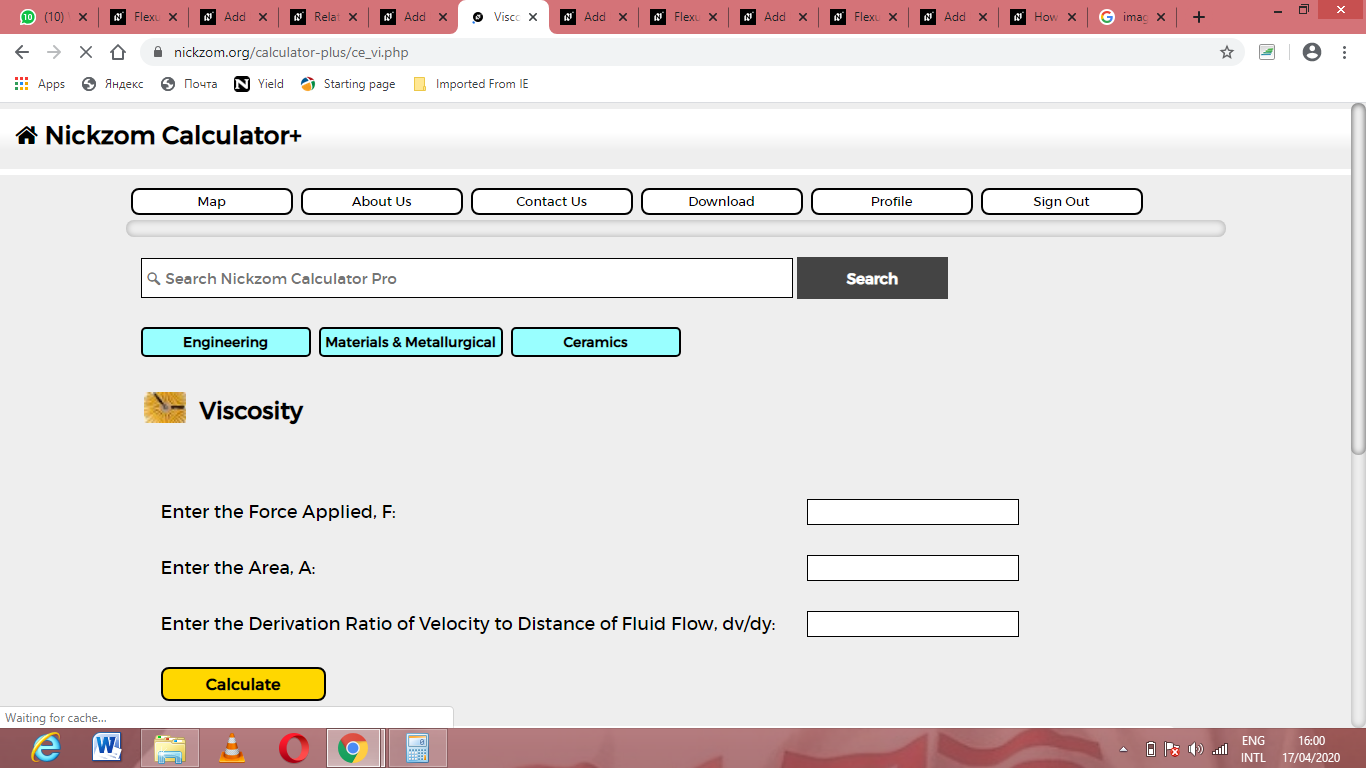Now, enter the values appropriately and accordingly for the parameters as required by the Force applied (F) is 21, Area (A) is 14 and Derivation Ratio of Velocity to Distance of Fluid Flow (dv/dy) is 19.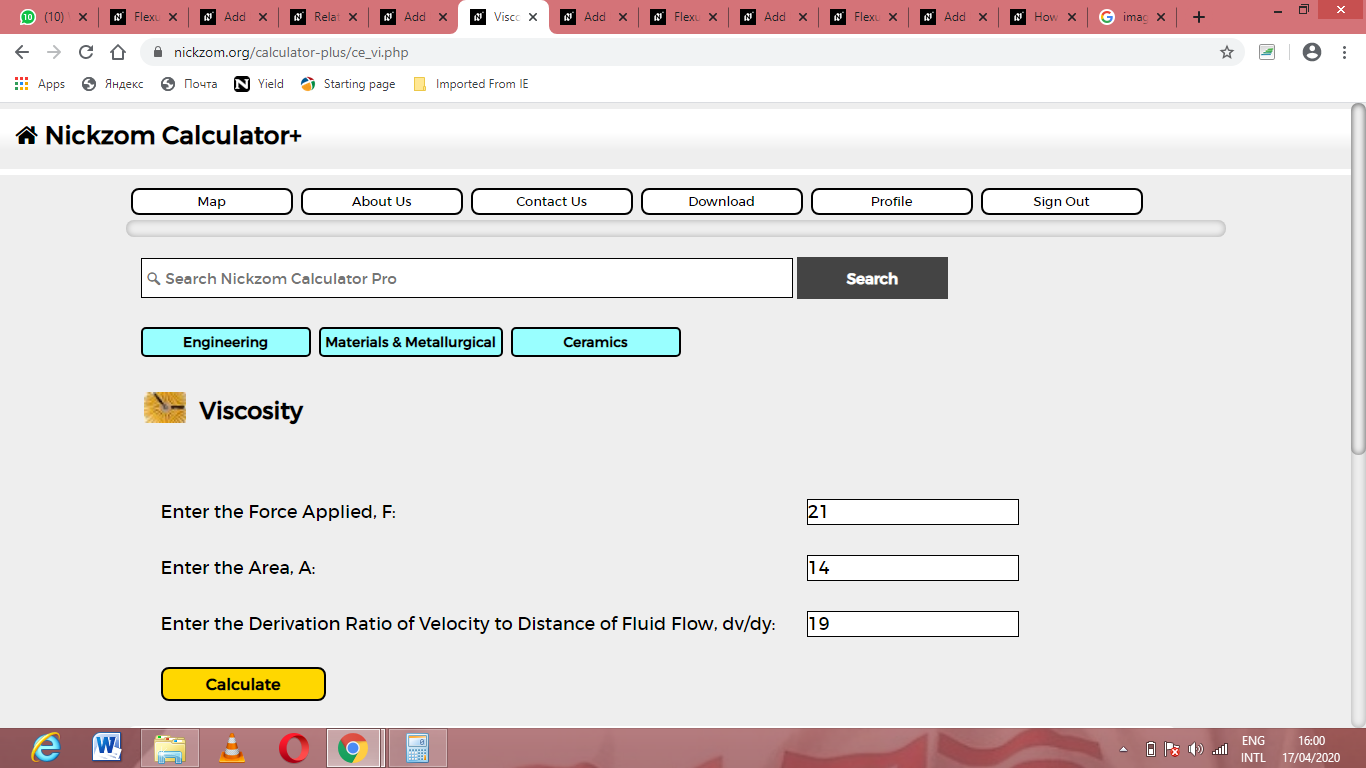Finally, Click on Calculate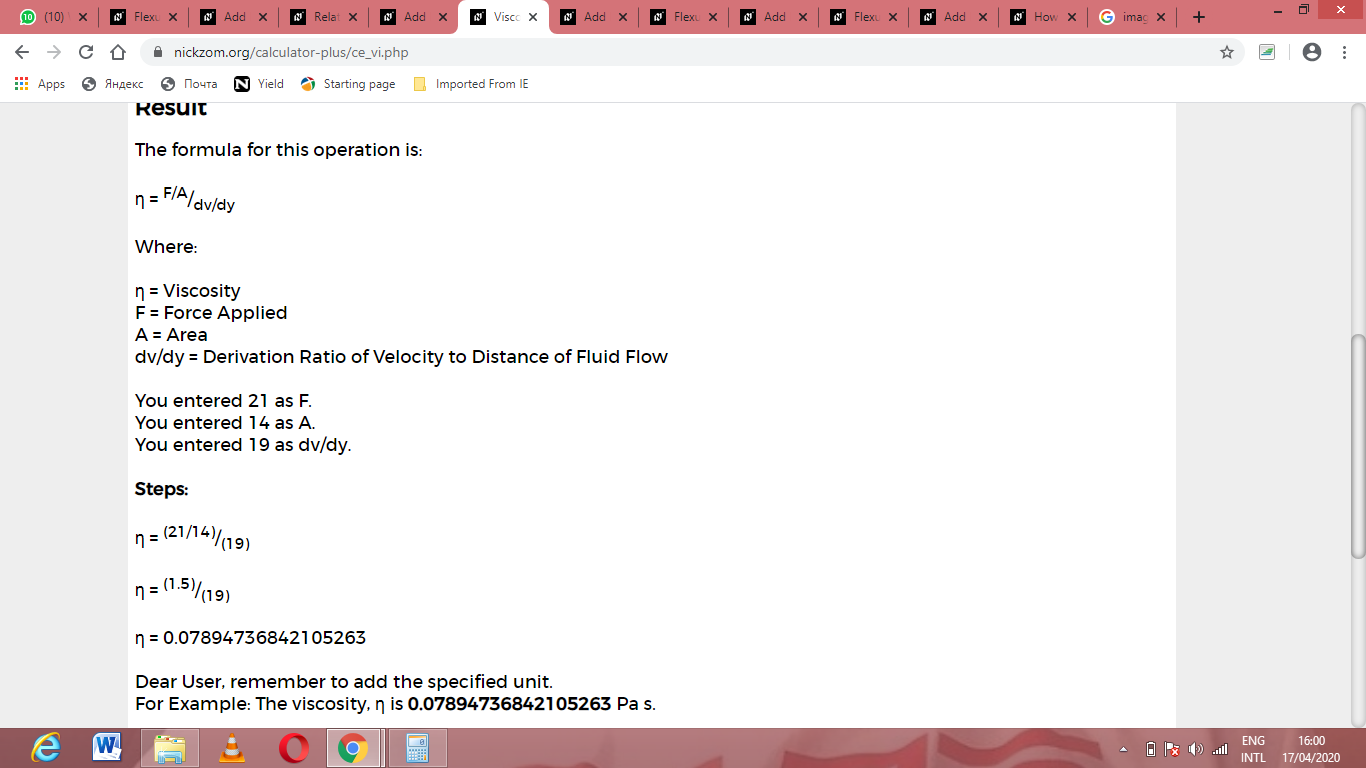As you can see from the screenshot above, Nickzom Calculator– The Calculator Encyclopedia solves for the viscosity and presents the formula, workings and steps too.